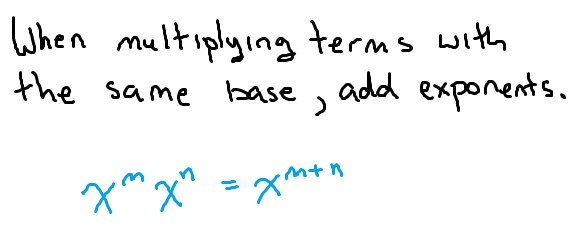# The laws of exponents

The laws of exponents (sometimes called the rules of exponents) allow us to simplify expressions by using our understanding of what an exponent means in much the same way that combining like terms uses the idea of what multiplication means. In both cases, the idea is to write algebraic expressions in the most simple way possible.

As a brief reminder, an exponent tells you how many times to multiply a number, variable or expression times itself. For example:$x^4=x \cdot x\cdot x\cdot x$$(x+5)^3=(x+5)(x+5)(x+5)$

All of the rules require that the terms have the SAME BASE. The base is whatever is being taken to a power. Above,$x$ is the base in the first example and$x+5$ is the base in the second example.

## Multiplying terms with exponents### Examples:

•$x^{9}x^{3}=x^{9+3}=x^{12}$
•$y^{4}y^{10}=y^{4+10}=y^{14}$

### Why does this work?

If we have$3^43^2$, this means to multiply$3^4$ by$3^2$. But,$3^4=3\cdot3\cdot3\cdot3$ while$3^2=3\cdot3$. Looking at the original example,$3^43^2 = (3\cdot3\cdot3\cdot3)(3\cdot3)$ and if you count the number of 3’s being multiplied, there are 6. So really,$3^43^2$ is a complicated way to write$3^6$.

## Dividing terms with exponents### Examples:

•$\dfrac{y^7}{y^4}=y^{7-4}=y^3$
•$\dfrac{x^2}{x^5}=x^{2-5}=x^{-3}=\dfrac{1}{x^3}$

### Why does this work?

As an example, think about$\dfrac{5^4}{5^2}$. This can be rewritten by writing out the multiplication$\dfrac{5\cdot5\cdot5\cdot5}{5\cdot5}$. At this point, we can cancel any factors that are shared by the numerator and the denominator and are left with$\dfrac{5\cdot5}{1}=5^2$. The “rule” is essentially doing this work for us by subtracting.

## Taking a “power to a power”### Examples:

•$(x^4)^4=x^{16}$
•$(-5x)^2=(-5)^2x^2=25x^2$

### Why does this work?

Let’s use another example:$(8^2)^3$. If an exponent tells us how many times to multiply something by itself, then this means to multiply$8^2$ by itself 3 times:$8^28^28^2$. Now, we have three terms with exponents multiplied that all have the same base. This is the first rule of exponents so we add them:$8^28^28^2 = 8^6$. This rule shortcuts this process.

## Other properties of exponents

When you begin simplifying expressions with exponents, there will more other properties you may find helpful.

• Anything to the 1 power is just the same term back again.$23^1=23$
• Anything other than zero the 0 power is 1.$(x+y+z^{12})^0=1$ (this is not true for$0^0$ which is an indeterminate form)
• Exponents distribute over multiplication and division only!$(xy)^2=x^{2}y^{2}$ but$(x+y)^2 \neq x^2 + y^2$. To find$(x+y)^2$ you must use FOIL.
• Negative exponents and fractional exponents have a specific meaning, which you can review here: http://www.mathbootcamps.com/understanding-exponents/.